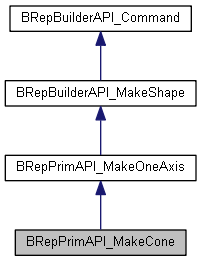# BRepPrimAPI_MakeCone Class Reference

Describes functions to build cones or portions of cones. A MakeCone object provides a framework for: More...

`#include <BRepPrimAPI_MakeCone.hxx>`

Inheritance diagram for BRepPrimAPI_MakeCone:[legend]

## Public Member Functions

BRepPrimAPI_MakeCone (const Standard_Real R1, const Standard_Real R2, const Standard_Real H)
Make a cone of height H radius R1 in the plane z = 0, R2 in the plane Z = H. R1 and R2 may be null. More...

BRepPrimAPI_MakeCone (const Standard_Real R1, const Standard_Real R2, const Standard_Real H, const Standard_Real angle)
Make a cone of height H radius R1 in the plane z = 0, R2 in the plane Z = H. R1 and R2 may be null. Take a section of <angle> More...

BRepPrimAPI_MakeCone (const gp_Ax2 &Axes, const Standard_Real R1, const Standard_Real R2, const Standard_Real H)
Make a cone of height H radius R1 in the plane z = 0, R2 in the plane Z = H. R1 and R2 may be null. More...

BRepPrimAPI_MakeCone (const gp_Ax2 &Axes, const Standard_Real R1, const Standard_Real R2, const Standard_Real H, const Standard_Real angle)
Make a cone of height H radius R1 in the plane z = 0, R2 in the plane Z = H. R1 and R2 may be null. Take a section of <angle> Constructs a cone, or a portion of a cone, of height H, and radius R1 in the plane z = 0 and R2 in the plane z = H. The result is a sharp cone if R1 or R2 is equal to 0. The cone is constructed about the "Z Axis" of either: More...

Returns the algorithm. More...

BRepPrim_ConeCone ()
Returns the algorithm. More...Public Member Functions inherited from BRepPrimAPI_MakeOneAxis
virtual void Build () override
Stores the solid in myShape. More...

const TopoDS_FaceFace ()
Returns the lateral face of the rotational primitive. More...

operator TopoDS_Face ()

const TopoDS_ShellShell ()
Returns the constructed rotational primitive as a shell. More...

operator TopoDS_Shell ()

const TopoDS_SolidSolid ()
Returns the constructed rotational primitive as a solid. More...

operator TopoDS_Solid ()Public Member Functions inherited from BRepBuilderAPI_MakeShape
virtual const TopoDS_ShapeShape ()
Returns a shape built by the shape construction algorithm. Raises exception StdFail_NotDone if the shape was not built. More...

operator TopoDS_Shape ()

virtual const TopTools_ListOfShapeGenerated (const TopoDS_Shape &S)
Returns the list of shapes generated from the shape <S>. More...

virtual const TopTools_ListOfShapeModified (const TopoDS_Shape &S)
Returns the list of shapes modified from the shape <S>. More...

virtual Standard_Boolean IsDeleted (const TopoDS_Shape &S)
Returns true if the shape S has been deleted. More...Public Member Functions inherited from BRepBuilderAPI_Command
virtual ~BRepBuilderAPI_Command ()

virtual Standard_Boolean IsDone () const

void Check () const
Raises NotDone if done is false. More...Protected Member Functions inherited from BRepBuilderAPI_MakeShape
BRepBuilderAPI_MakeShape ()Protected Member Functions inherited from BRepBuilderAPI_Command
BRepBuilderAPI_Command ()
Set done to False. More...

void Done ()
Set done to true. More...

void NotDone ()
Set done to false. More...Protected Attributes inherited from BRepBuilderAPI_MakeShape
TopoDS_Shape myShape

TopTools_ListOfShape myGenerated

## Detailed Description

Describes functions to build cones or portions of cones. A MakeCone object provides a framework for:

• defining the construction of a cone,
• implementing the construction algorithm, and
• consulting the result.

## ◆ BRepPrimAPI_MakeCone() [1/4]

 BRepPrimAPI_MakeCone::BRepPrimAPI_MakeCone ( const Standard_Real R1, const Standard_Real R2, const Standard_Real H )

Make a cone of height H radius R1 in the plane z = 0, R2 in the plane Z = H. R1 and R2 may be null.

## ◆ BRepPrimAPI_MakeCone() [2/4]

 BRepPrimAPI_MakeCone::BRepPrimAPI_MakeCone ( const Standard_Real R1, const Standard_Real R2, const Standard_Real H, const Standard_Real angle )

Make a cone of height H radius R1 in the plane z = 0, R2 in the plane Z = H. R1 and R2 may be null. Take a section of <angle>

## ◆ BRepPrimAPI_MakeCone() [3/4]

 BRepPrimAPI_MakeCone::BRepPrimAPI_MakeCone ( const gp_Ax2 & Axes, const Standard_Real R1, const Standard_Real R2, const Standard_Real H )

Make a cone of height H radius R1 in the plane z = 0, R2 in the plane Z = H. R1 and R2 may be null.

## ◆ BRepPrimAPI_MakeCone() [4/4]

 BRepPrimAPI_MakeCone::BRepPrimAPI_MakeCone ( const gp_Ax2 & Axes, const Standard_Real R1, const Standard_Real R2, const Standard_Real H, const Standard_Real angle )

Make a cone of height H radius R1 in the plane z = 0, R2 in the plane Z = H. R1 and R2 may be null. Take a section of <angle> Constructs a cone, or a portion of a cone, of height H, and radius R1 in the plane z = 0 and R2 in the plane z = H. The result is a sharp cone if R1 or R2 is equal to 0. The cone is constructed about the "Z Axis" of either:

• the global coordinate system, or
• the local coordinate system Axes. It is limited in these coordinate systems as follows:
• in the v parametric direction (the Z coordinate), by the two parameter values 0 and H,
• and in the u parametric direction (defined by the angle of rotation around the Z axis), in the case of a portion of a cone, by the two parameter values 0 and angle. Angle is given in radians. The resulting shape is composed of:
• a lateral conical face
• two planar faces in the planes z = 0 and z = H, or only one planar face in one of these two planes if a radius value is null (in the case of a complete cone, these faces are circles), and
• and in the case of a portion of a cone, two planar faces to close the shape. (either two parallelograms or two triangles, in the planes u = 0 and u = angle). Exceptions Standard_DomainError if:
• H is less than or equal to Precision::Confusion(), or
• the half-angle at the apex of the cone, defined by R1, R2 and H, is less than Precision::Confusion()/H, or greater than (Pi/2)-Precision::Confusion()/H.f

## ◆ Cone()

 BRepPrim_Cone& BRepPrimAPI_MakeCone::Cone ( )

Returns the algorithm.